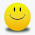Latest Articles

# Finding Squares of any Numbers easy and effective

Info Post
A simple trick to find the square of a number that is not closer to 10, 100, 1000 .

Find the square if 34 ??

First ,
Add the number to the second digit = 34+4= 38 --- (1)
Multiply the first digit of the number with number obtained in (1)
i.e ;  3 x 38 =114. This is the first part of the answer.
Square the last digit = 4 x 4

Answer = First part | Last part
=  114 | 16
= 1156

Therefore,34 2 = 1156

Diagrammatic Representation

Squaring of Numbers close to the powers of base 10, 100, 1000 etc
Squaring of Numbers close to base 50
To Multiply any number by any number
Share:

1.